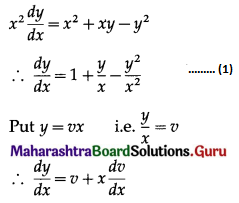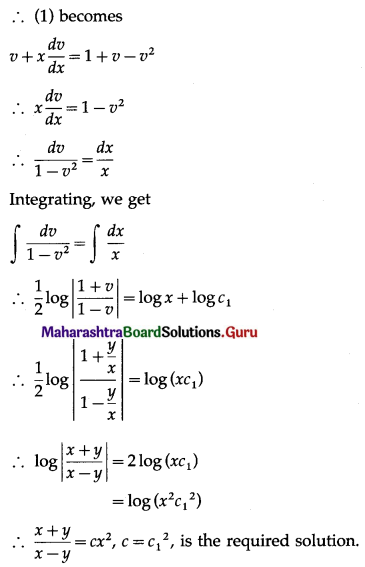# Maharashtra Board 12th Commerce Maths Solutions Chapter 8 Differential Equation and Applications Ex 8.4

Balbharati Maharashtra State Board Std 12 Commerce Statistics Part 1 Digest Pdf Chapter 8 Differential Equation and Applications Ex 8.4 Questions and Answers.

## Maharashtra State Board 12th Commerce Maths Solutions Chapter 8 Differential Equation and Applications Ex 8.4

Solve the following differential equations:

Question 1.
x dx + 2y dy = 0
Solution:
x dx + 2y dy = 0
Integrating, we get
∫x dx + 2 ∫y dy = c1
∴ $$\frac{x^{2}}{2}+2\left(\frac{y^{2}}{2}\right)=c_{1}$$
∴ x2 + 2y2 = c, where c = 2c1
This is the general solution.Question 2.
y2 dx + (xy + x2) dy = 0
Solution:
y2 dx + (xy + x2) dy = 0
∴ (xy + x2) dy = -y2 dx
∴ $$\frac{d y}{d x}=\frac{-y^{2}}{x y+x^{2}}$$ ………(1)
Put y = vx
∴ $$\frac{d y}{d x}=v+x \frac{d v}{d x}$$
Substituting these values in (1), we get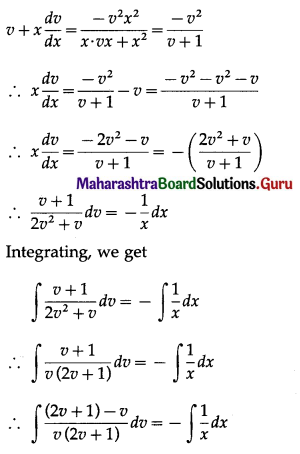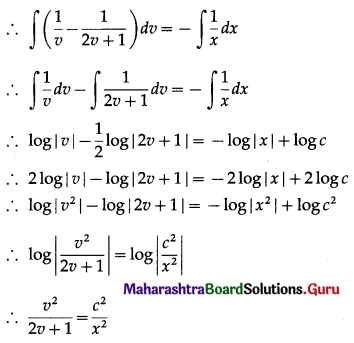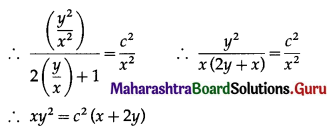This is the general solution.

Question 3.
x2y dx – (x3 + y3) dy = 0
Solution:
x2y dx – (x3 + y3) dy = 0
∴ (x3 + y3) dy = x2y dx
∴ $$\frac{d y}{d x}=\frac{x^{2} y}{x^{3}+y^{3}}$$ ……(1)
Put y = vx
∴ $$\frac{d y}{d x}=v+x \frac{d v}{d x}$$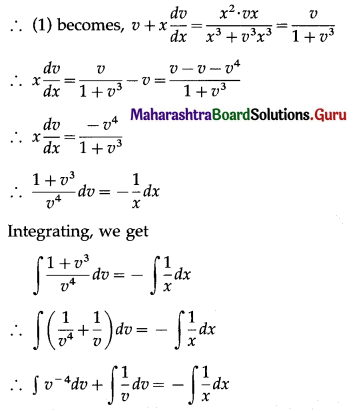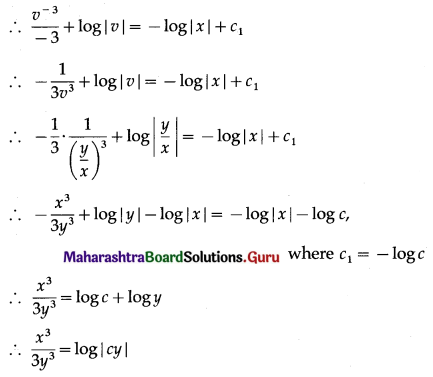This is the general solution.Question 4.
$$\frac{d y}{d x}+\frac{x-2 y}{2 x-y}=0$$
Solution: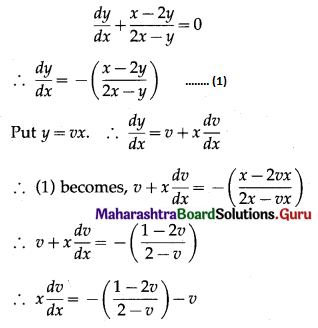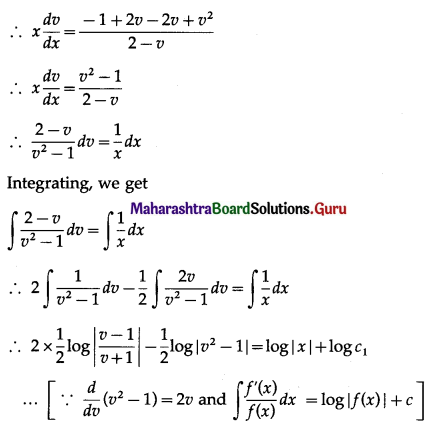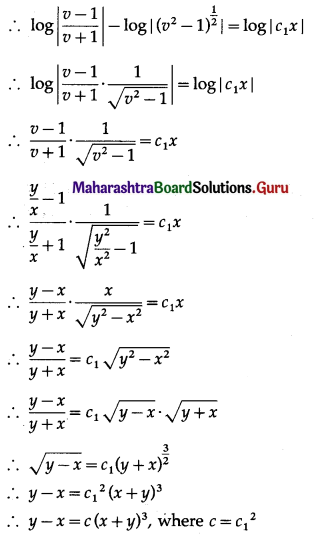This is the general solution.

Question 5.
(x2 – y2) dx + 2xy dy = 0
Solution:
(x2 – y2) dx + 2xy dy = 0
∴ 2xy dy = -(x2 – y2) dx = (y2 – x2) dx
∴ $$\frac{d y}{d x}=\frac{y^{2}-x^{2}}{2 x y}$$ ………(1)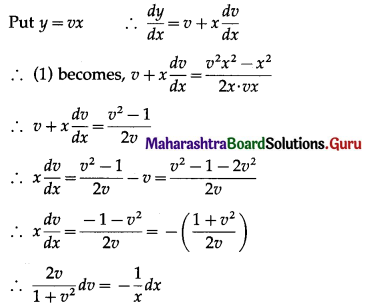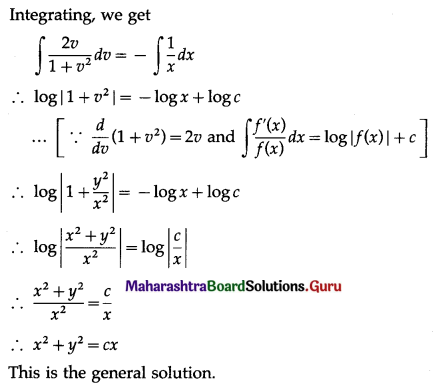Question 6.
xy$$\frac{d y}{d x}$$ = x2 + 2y2
Solution: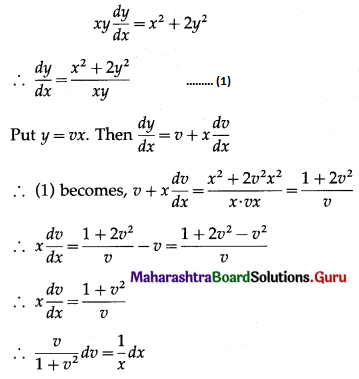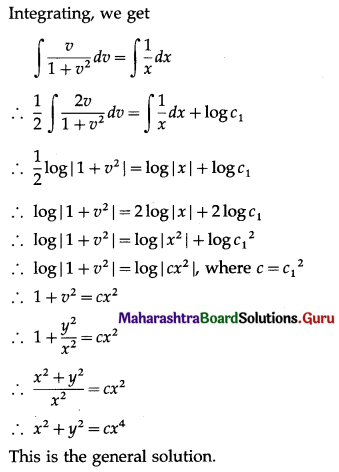Question 7.
x2$$\frac{d y}{d x}$$ = x2 + xy – y2
Solution: